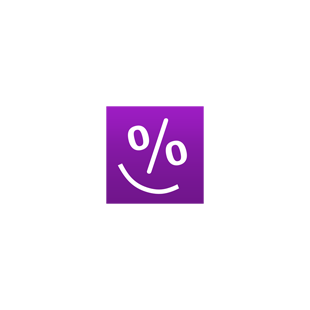Original price was \$9.99, current price \$4.99
50% off

Overview System Requirements Related

Description

Smart Percentage Calculator is a must-have app for everyday calculations - simple percentage calculations, percentage increase/decrease, VAT calculations, calculating tips, sale price, percentage off, discounted price, and more. FEATURES: • SIMPLE PERCENTAGE: - finds the percentage of a value (what is 5% of 20?) - finds the value from a percentage - finds the whole number when you know the percentage and the sub-value (if 4 is 30%, what is the whole number?) - sub-value of a value - finds the proportion of one number to another in percentage terms (20 is what percent of 150?) • ADD OR SUBTRACT A PERCENTAGE • PERCENTAGE CHANGE Finds the percentage change (increase/decrease) between two values. • FRACTION TO PERCENTAGE Converts fractions to percentages and percentages to fractions. • VAT - calculates the price without VAT (net price). - calculates the price with VAT (gross price). - calculates the VAT amount. • DISCOUNT - calculate the discounted price and your saving - handles multiple discounts Smart Percentage Calculator makes percentage calculations based on various inputs including values, sub-values, percentages, ratios, fractions, percentage changes, percentage increase/decrease. If any two of values are entered, the calculator finds the third one. For VAT calculations you may enter any VAT rate. Discount calculations: just enter the original price, discount, sales tax percentage (if applicable) and get the final price and the amount of money you save.You can enter discount percentage or discount amount. You can select rounding to the specified number of decimal places from 0 to 3. By default, the app displays 2 decimal places. To round results to the nearest whole number, select 0 decimal places. Supports fractional values. You can make calculations in one of 15 supported currencies. History tape to view recent calculations (25 entries). Back and forward buttons lets you view your recent calculations. Share results and history via email. 'Undo' for the Clear command. Supports both portrait and landscape orientation.

Screenshots

Intemodino Group s.r.o.

Intemodino Group

9/23/2017

26.82 MB

For all ages

This app can

Access all your files, peripheral devices, apps, programs and registry
Microsoft.storeFilter.core.notSupported_8wekyb3d8bbwe

Installation

Get this app while signed in to your Microsoft account and install on up to ten Windows 10 devices.

Language supported

English (United States)
Čeština (Česká Republika)
Русский (Россия)
Deutsch (Deutschland)
Français (France)
Español (España, Alfabetización Internacional)
Polski (Polska)
Português (Brasil)
Dansk (Danmark)
Nederlands (Nederland)
Italiano (Italia)
Suomi (Suomi)
Ελληνικά (Ελλάδα)
Türkçe (Türkiye)

한국어(대한민국)
Norsk Bokmål (Norge)
Svenska (Sverige)